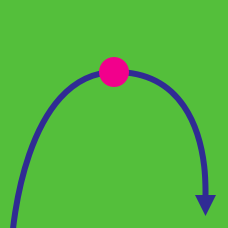Calculus

# Concave / Convex Functions

What is the inflection point of the curve $y=x^3-3x^2+7x+12?$

What is the maximum value of real number $a$ such that the curve $y=x^2(\ln x-13)$ is concave down in the interval $(0,a)?$

If $f(x) = \ln (x^2 + 9)$, the interval on which the curve $y = f(x)$ is concave up can be expressed as $a < x < b$. What is the value of $b - a$?

If $f(x) = x^4 - 32 x^3 + 288 x^2 + 8x - 15$, the interval on which the curve $y = f(x)$ is concave down can be expressed as $a < x < b$. What is the value of $a + b$?

Let $f(x) = x^4 - 40 x^3 + 504x^2 + 19x + 17$. Given that $f(x)$ is concave in the domain $\left[a,b\right]$, what is the value of $a+b$?

×